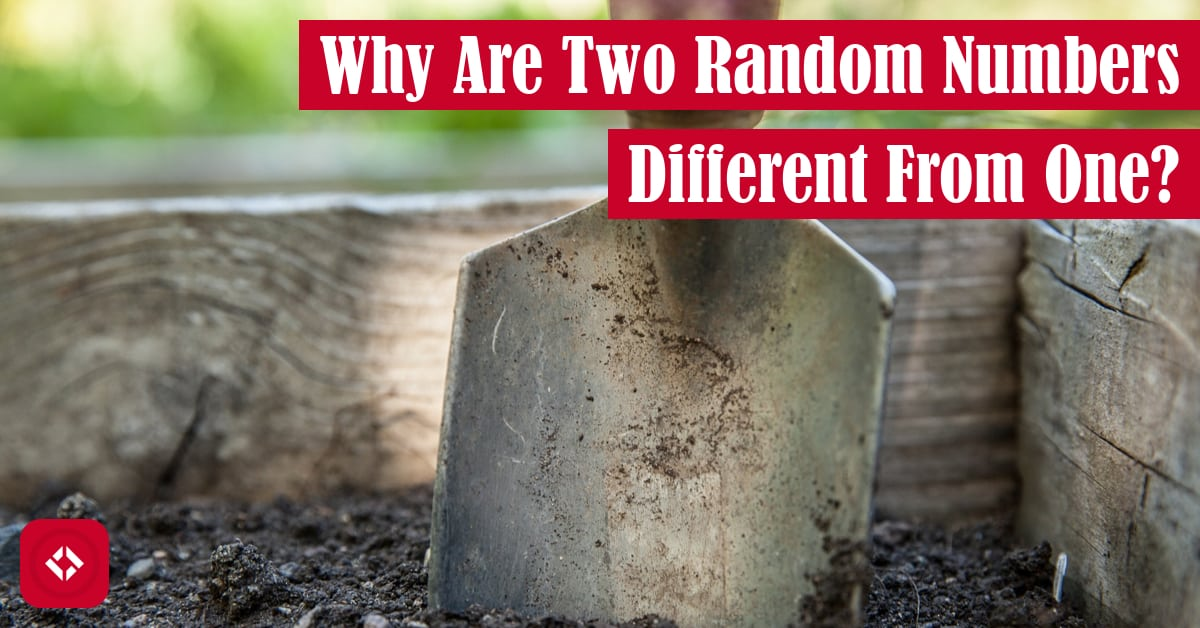# Why Is Adding Two Random Numbers Not the Same as Generating One in the Same Range?This may seem like an oddly specific article, but it gets at a problem that a lot of folks struggle with when generating random numbers: why shouldn’t I just add two random numbers together? Why does it make more sense to generate one? That’s the topic of today’s article!

## Introducing the Problem

In one of the courses I teach, we have a sample exam problem that reads as follows:

Suppose you want to set the double variable oneToThree to a random real number
uniformly distributed in the interval [1.0, 3.0). You have made the following
declaration:

`Random r = new Random1L();`

noting that `r.nextDouble()` returns a random real number uniformly distributed in
the interval [0.0, 1.0). Which statement will set `oneToThree` to the desired result?

SourceThe proposed answers to this question are as follows:

1. `oneToThree = 3.0 * r.nextDouble();`
2. `oneToThree = 1.0 + 2.0 * r.nextDouble();`
3. `oneToThree = 1.0 + r.nextDouble() + r.nextDouble();`
4. `oneToThree = r.nextDouble() + r.nextDouble() + r.nextDouble();`

Most of the time, students will be able to work there way down to two of the possible answers: 2 and 3. However, they get stuck because they realize that the ranges for both end up being [1.0, 3.0). And in fact, this had troubled me for some time as well. Until one day, a student actually helped me explain the difference, so today I want to pass that knowledge off to you.

## Simplifying the Problem

One way that I like to explain the difference between generating a single number in the correct range and adding a pair of numbers in the correct range is through an example with dice. Most of us are familiar with the typical six-sided die. Alone, they can generate a number between 1 and 6. In a pair, the range changed to 2 to 12, a set of 11 possible numbers. If we imagine we have an 11-sided die with an equivalent range, 2 to 11, will we get the same behavior? The answer is no.

While the ranges are the same, the probability distributions are different. On the 11-sided die, any number is equally possible. When we toss two six-sided dice, however, not every sum is equally likely. In fact, here’s a table of all the ways the dice can be rolled to produce a particular number:

Part of the reason I chose to show the combination in this way is you can literally visualize the probability distribution. When two dice are rolled, despite having the same range, the distribution is drastically different. Specifically, numbers near the middle of the range are significantly more likely than the values at the extremes. In contrast, on an 11-sided die, 2 and 12 are just as likely as 7.

Tying this idea back to our random number generator example, it should be more clear how the two solutions have different probability distributions. Specifically, generating two random numbers causes a distribution like that of rolling a pair of dice. But of course, you shouldn’t just believe me. Let’s try to show it empirically.

## Demonstrating the Problem

Because I’m a Python elitist, I’m going to put together a quick script to show the difference in distribution using the original example:

```import random

def oneToThreeDie():
return 1 + 2.0 * random.random()

def oneToThreeDice():
return 1 + random.random() + random.random()

def buckets(data):
dieBuckets = [0, 0, 0]
for num in data:
if num < 1.666:
dieBuckets += 1
elif 1.666 < num <= 2.333:
dieBuckets += 1
else:
dieBuckets += 1
return dieBuckets

die = [oneToThreeDie() for _ in range(100000)]
dice = [oneToThreeDice() for _ in range(100000)]

print(buckets(die))
print(buckets(dice))```

Here, you should notice that there are two functions mapping both of our random number generators: die and dice. The die method generates a uniformly distributed number between 1 and 3. Meanwhile, the dice method generates a number along the same range with a distribution that tends toward the middle.

To prove the difference in distributions, I wrote the buckets method which takes a list of numbers and sorts them into buckets. Each list of numbers is generated using a list comprehension and the appropriate number generating function.

When run, the program prints out two lists of three items. These lists are meant to segment the distribution into three somewhat equal parts (i.e., 1 to 1.666, 1.666 to 2.333, and 2.333 to 3). If the distributions are truly uniform, we should see roughly equal numbers of generated terms in each bucket. If, however, the middle bucket contains more terms, then the distribution is not uniform. Unsurprisingly, when I run this script, I get the following results:

```[33156, 33325, 33519]
[22160, 55784, 22056]```

The first list refers to the generation of a single number, and the second list refers to the generation of a pair of numbers. As you can see, when two numbers are generated, it’s actually about twice as likely for the sum to be in the middle third of the distribution than along the outer thirds.

This, of course, begs the question: if we generate more numbers, how is the distribution affected? That’s not a question I’m looking to answer today, but I would definitely be interested in exploring more in the future, especially with a bit of data visualization. With that said, that’s about all I want to cover for now. As always, if you liked this and want to read more like it, check out one of the following articles:

Otherwise, you might consider some of the following resources (#ad):

If not, take care! I hope to see you again soon.